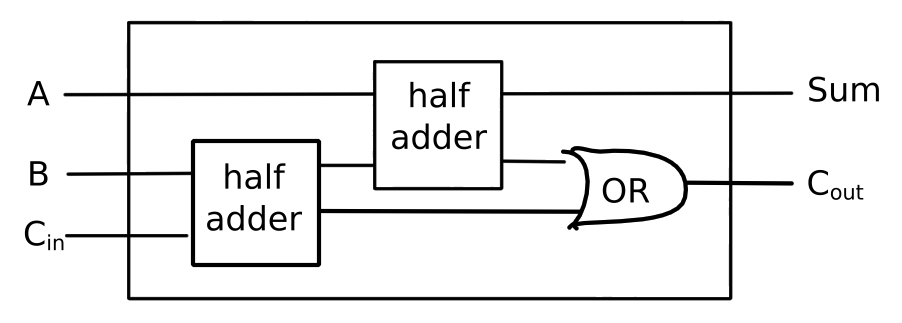# SICP 5b Computational Objects

## 1 Electrical systems

To understand how to build correspondences between objects in the world, and objects in the computer, let's take the example of electrical systems.

Primitive and means of combination

```(define a (make-wire))
(define b (make-wire))
(define c (make-wire))
(define d (make-wire))
(define e (make-wire))
(define s (make-wire))

(or-gate a b d)
(and-gate a b c)
(inverter c e)
(and-gate d e s)
```

Means of abstraction

```(define (half-adder a b s c)
(let ((d (make-wire)) (e (make-wire)))
(or-gate a b d)
(and-gate a b c)
(inverter c e)
(and-gate d e s)))
```

すじにくシチュー, published under Creative Commons CC0 1.0 Universal Public Domain Dedication

Lambda is the ultimate glue.

```(define (full-adder a b c-in sum c-out)
(let ((s (make-wire))
(c1 (make-wire))
(c2 (make-wire)))
(or-gate c1 c2 c-out)))
```As the means of combination and abstraction are inherited from LISP, only the primitives need to be implemented.

```(define (inverter in out)
(define (invert-in)
(let ((new
(logical-not (get-signal in))))
(after-delay inverter-delay
(lambda ()
(set-signal! out new)))))

(define (logical-not s)
(cond ((= s 0) 1)
((= s 1) 0)
(else
(error "invalid signal" s))))

(define (and-gate a1 a2 output)
(define (and-action-procedure)
(let ((new-value
(logical-and (get-signal a1)
(get-signal a2))))
(after-delay and-gate-delay
(lambda ()
(set-signal! output
new-value)))))

(define (make-wire)
(let ((signal 0) (action-procs '()))
(define (set-my-signal! new)
(cons ((= signal new) 'done)
(else
(set! signal new)
(call-each action-procs))))
(define (accept-action-proc proc)
(set! action-procs
(cons proc action-procs))
(proc))
(define (dispatch m)
(cond ((eq? m 'get-signal) signal)
((eq? m 'set-signal!)
set-my-signal!)
accept-action-proc)
(else
dispatch))

(define (call-each procedures)
(cond ((null? procedures) 'done)
(else
((car procedures))
(call-each (cdr procedures)))))

(define (get-signal wire)
(wire 'get-signal))

(define (set-signal! wire new-value)
((wire 'set-signal!) new-value))

(define (after-delay delay action)
(+ delay (current-time the-agenda))
action
the-agenda))

(define (propagate)
(cond ((empty-agenda? the-agenda)
'done)
(else
((first-item the-agenda))
(remove-first-item! the-agenda)
(propagate))))
```

Now let's see the machinery in action.

```(define the-agenda (make-agenda))
(define inverter-delay 2)
(define and-gate-delay 3)
(define or-gate-delay 5)

(define input-1 (make-wire))
(define input-2 (make-wire))
(define sum (make-wire))
(define carry (make-wire))

(probe 'sum sum)
sum 0 new-value = 0
(probe 'carry carry)
carry 0 new-value = 0
```

A `probe` is an object that has the property that when you change a wire that it's attached to, it types out a message.

```(half-adder input-1 input-2 sum carry)
(set-signal! input-1 1)
(propagate)
sum 8 new-value = 1
done
(set-signal input-2 1)
(propagate)
carry 11 new-value = 1
sum 16 new-value = 0
done
```

## 2 Agendas and queues

What functionality is required from agendas and queues?

Agendas

```(make-agenda)
(current-time agenda)
(empty-agenda? agenda)
(first-item agenda)
(remove-first-item agenda)
```

Agendas consist of a header, followed by segments comprised of pairs of times and queues.

Queues

```(make-queue)
(insert-queue! queue item)
(delete-queue! queue)
(front-queue queue)
(empty-queue? queue)
```

In order to insert a new segment in an agenda between the first and last item, the front and rear have to be changed. Hence the following procedures are required.

```(set-car! <pair> <value>)
(set-cdr! <pair> <value>)
```

## 3 Danger with lists

With the implementation of `set!`, care needs to be taken with lists, as elements within can be changed.

```(define a (cons 1 2))
(define b (cons a a))
(set-car! (car b) 3)
(car a)
```
```3
```
```(cadr b)
```
```3
```

It is impossible to mitigate this. If `set!` is allowed, so are `set-car!`, `set-cdr!` etc.

Once you let the camel's nose into the tent, the rest of him follows.

## 4 Church of Alonzo

`cons` can be constructed out of hot air. The idea was originally concocted by Alonzo Church, the greatest programmer of the 20th century (even though he never saw a computer).

```(define (cons x y)
(lambda (m) (m x y)))
(define (car x)
(x (lambda (a d) a)))
(define (cdr x)
(x (lambda (a d) d)))
```

Surely this doesn't work? A step-by-step example must surely prove this to be nonsense…

```(car (cons 35 47))
(car (lambda (m) (m 35 47)))
((lambda (m) (m 35 47)) (lambda (a d) a))
((lambda (a d) a) 35 47)
35
```

Marry this idea with mutable data.

```(define (cons x y)
(lambda (m)
(m x
y
(lambda (n) (set! x n))
(lambda (n) (set! y n)))))
(define (car x)
(x (lambda (a d sa sd) a)))
(define (cdr x)
(x (lambda (a d sa sd) d)))
(define (set-car! x y)
(x (lambda (a d sa sd) (sa y))))
(define (set-cdr! x y)
(x (lambda (a d sa sd) (sd y))))
```

Once I've introduced one assignment operator, I have introduced them all!